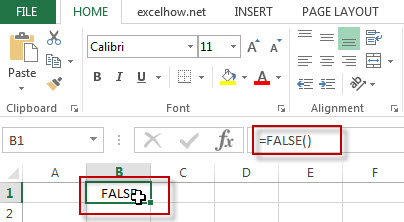# Excel False Function

This post will guide you how to use Excel FALSE function with syntax and examples in Microsoft excel.

### Description

The Excel FALSE function returns the logical value “FALSE”.

The FALSE function is a build-in function in Microsoft Excel and it is categorized as a Logical Function.

The FALSE function is available in Excel 2016, Excel 2013, Excel 2010, Excel 2007, Excel 2003, Excel XP, Excel 2000, Excel 2011 for Mac.

### Syntax

The syntax of the FALSE function is as below:

`=FALSE()`

### Example

The below examples will show you how to use Excel FALSE Function to return the logical value FALSE.

#1 To get False value in B1 Cell, just using the following excel formula: =FALSE()Related Functions

• Excel TRUE function
The Excel TRUE function return the logical value TRUE. And you can use the TRUE function when you want to return the TRUE value. For example, the formula: =IF(B1>5, TRUE()).The syntax of the TRUE function is as below:=TRUE ()…